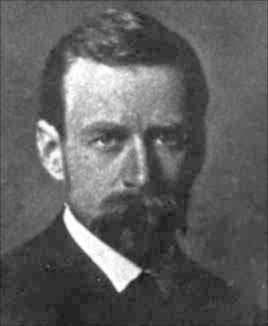# KOCH'S SNOWFLAKE

by Emily FungThe Koch Snowflake was created by the Swedish mathematician Niels Fabian Helge von Koch.In his 1904 paper entitled "Sur une courbe continue sans tangente, obtenue par une construction géométrique élémentaire" he used the Koch Snowflake to show that it is possible to have figures that are continuous everywhere but differentiable nowhere.

## The Koch Curve

In order to create the Koch Snowflake, von Koch began with the development of the Koch Curve. The Koch Curve starts with a straight line that is divided up into three equal parts. Using the middle segment as a base, an equilateral triangle is created. Finally, the base of the triangle is removed, leaving us with the first iteration of the Koch Curve.

## The Koch Snowflake

From the Koch Curve, comes the Koch Snowflake. Instead of one line, the snowflake begins with an equilateral triangle. The steps in creating the Koch Curve are then repeatedly applied to each side of the equilateral triangle, creating a "snowflake" shape.

The Koch Snowflake is an example of a figure that is self-similar, meaning it looks the same on any scale. In this picture the part of the figure in the red box is similar to the entire picture.

## The Math Behind It

NUMBER OF SIDES (n)

For each iteration, one side of the figure from the previous stage becomes four sides in the following stage. Since we begin with three sides, the formula for the number of sides in the Koch Snowflake is

n = 3*4a

in the ath iteration.

For iterations 0, 1, 2 and 3, the number of sides are 3, 12, 48 and 192, respectively.

LENGTH OF A SIDE (length)

In every iteration, the length of a side is 1/3 the length of a side from the preceding stage. If we begin with an equilateral triangle with side length x, then the length of a side in interation a is

length = x*3-a

For iterations 0 to 3, length = a, a/3, a/9 and a/27.

PERIMETER (p)

Since all the sides in every iteration of the Koch Snowflake is the same the perimeter is simply the number of sides multiplied by the length of a side

p = n*length

p = (3*4a)*(x*3-a)

for the ath iteration.

Again, for the first 4 iterations (0 to 3) the perimeter is 3a, 4a, 16a/3, and 64a/9.

As we can see, the perimeter increases by 4/3 times each iteration so we can rewrite the formula as

p = (3a)*(4/3)a

So as a-->infinity the perimeter continues to increase with no bound.

Also, as a-->infinity the snowflake is made up of sharp corners with no smooth lines connecting them. Therefore, while the perimeter of the snowflake, which is an infinite series, is continuous because there are no breaks in the perimeter, it is not differentiable since there are no smooth lines.

REFERENCES:

Eric W. Weisstein. "Koch Snowflake." From MathWorld--A Wolfram Web Resource. http://mathworld.wolfram.com/KochSnowflake.html

Tom VanCourt. "Glencoe's Manual of Fuzz." The Textbook League. http://www.textbookleague.org/102fuzz.htm

"Niels Fabian Helge Von Koch." School of Mathematics and Statistics. University of St. Andrews, Scotland. http://www-groups.dcs.st-and.ac.uk/~history/Mathematicians/Koch.html